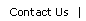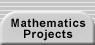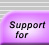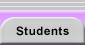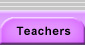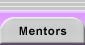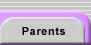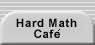# Euclid's Algorithm

Euclid's Algorithm is a procedure for finding the greatest common divisor (gcd) of a pair of numbers.

For a fun introduction that helps you gain an intuitive understanding of the algorithm, begin with Euclid's Game.

You can then read more at Euclid's Algorithm, the Extension of Euclid's Algorithm, and Notes on Greatest Common Divisors.Translations of mathematical formulas for web display were created by tex4ht. © Copyright 2003 Education Development Center, Inc. (EDC)